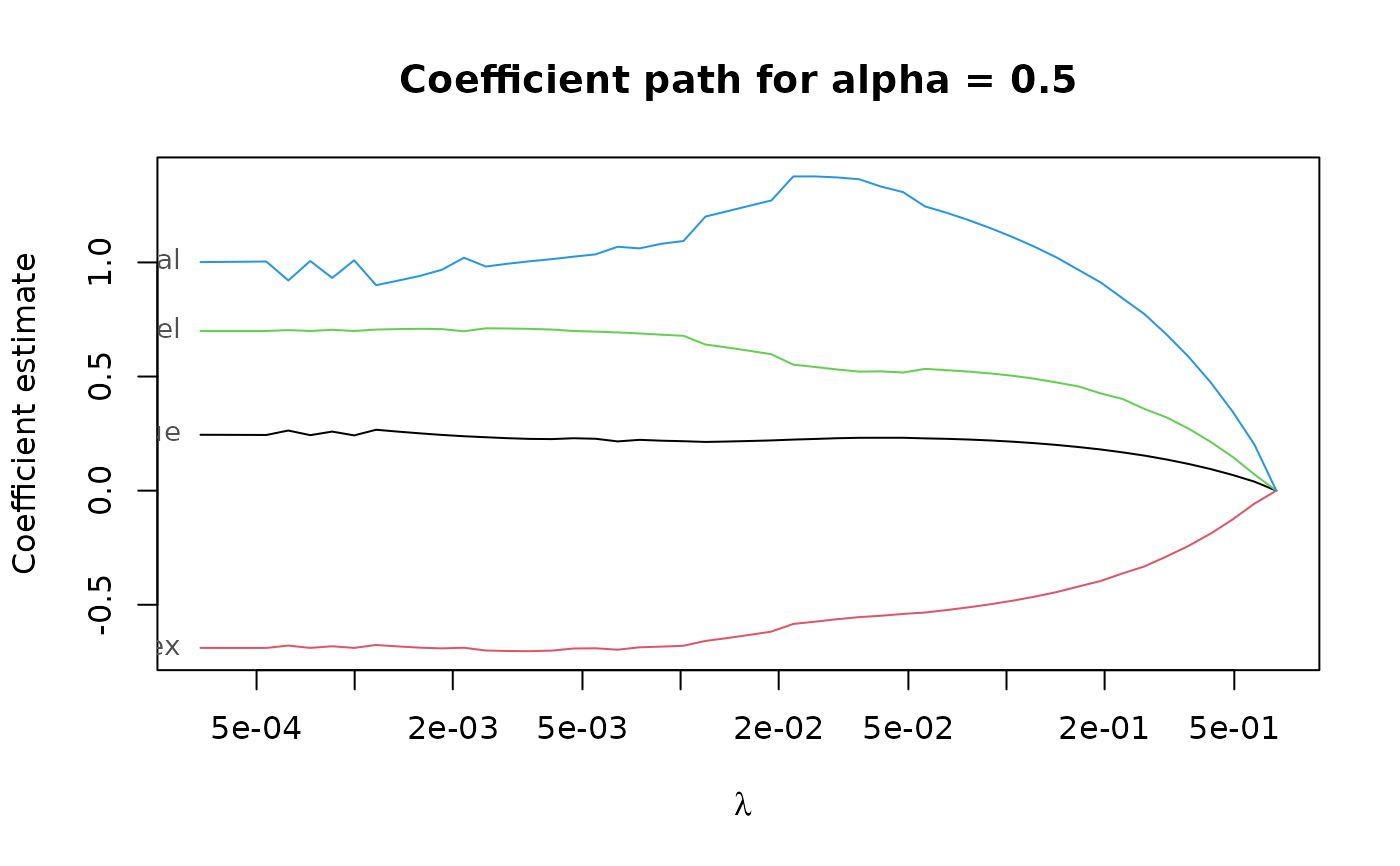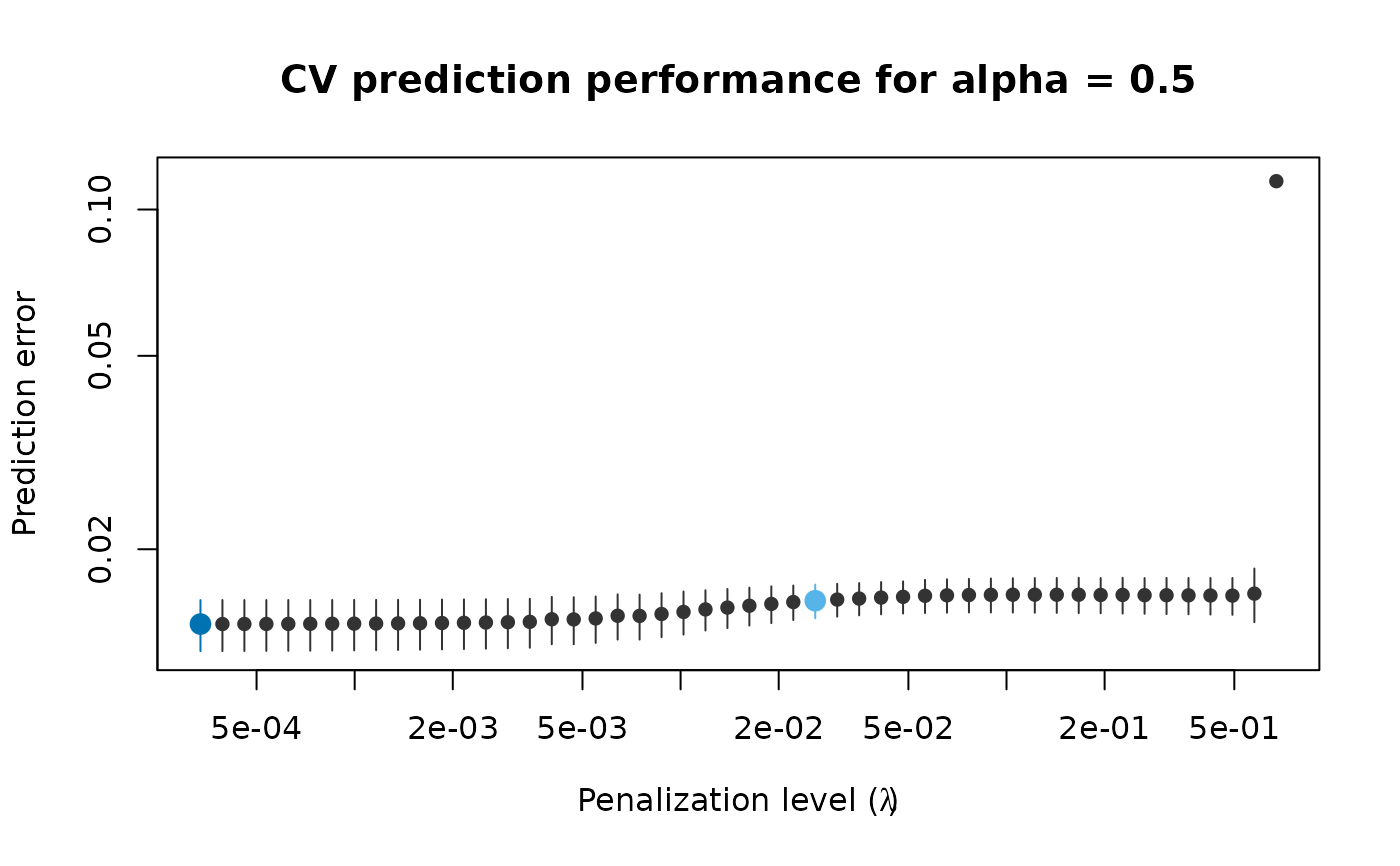Extract coefficients from an adaptive PENSE (or LS-EN) regularization path with hyper-parameters chosen by cross-validation.

# S3 method for pense_cvfit
coef(
object,
alpha = NULL,
lambda = "min",
se_mult = 1,
sparse = NULL,
standardized = FALSE,
exact = deprecated(),
correction = deprecated(),
...
)

object PENSE with cross-validated hyper-parameters to extract coefficients from. Either a single number or NULL (default). If given, only fits with the given alpha value are considered. If lambda is a numeric value and object was fit with multiple alpha values and no value is provided, the first value in object$alpha is used with a warning. either a string specifying which penalty level to use ("min", "se", "{m}-se") or a single numeric value of the penalty parameter. See details. If lambda = "se", the multiple of standard errors to tolerate. should coefficients be returned as sparse or dense vectors? Defaults to the sparsity setting of the given object. Can also be set to sparse = 'matrix', in which case a sparse matrix is returned instead of a sparse vector. return the standardized coefficients. defunct. currently not used. ## Value either a numeric vector or a sparse vector of type dsparseVector of size $$p + 1$$, depending on the sparse argument. Note: prior to version 2.0.0 sparse coefficients were returned as sparse matrix of type dgCMatrix. To get a sparse matrix as in previous versions, use sparse = 'matrix'. ## Hyper-parameters If lambda = "{m}-se" and object contains fitted estimates for every penalization level in the sequence, use the fit the most parsimonious model with prediction performance statistically indistinguishable from the best model. This is determined to be the model with prediction performance within m * cv_se from the best model. If lambda = "se", the multiplier m is taken from se_mult. By default all alpha hyper-parameters available in the fitted object are considered. This can be overridden by supplying one or multiple values in parameter alpha. For example, if lambda = "1-se" and alpha contains two values, the "1-SE" rule is applied individually for each alpha value, and the fit with the better prediction error is considered. In case lambda is a number and object was fit for several alpha hyper-parameters, alpha must also be given, or the first value in object$alpha is used with a warning.

Other functions for extracting components: coef.pense_fit(), predict.pense_cvfit(), predict.pense_fit(), residuals.pense_cvfit(), residuals.pense_fit()

## Examples

# Compute the PENSE regularization path for Freeny's revenue data
# (see ?freeny)
data(freeny)
x <- as.matrix(freeny[ , 2:5])

regpath <- pense(x, freeny$y, alpha = 0.5) plot(regpath)# Extract the coefficients at a certain penalization level coef(regpath, lambda = regpath$lambda[][])
#>           (Intercept) lag.quarterly.revenue           price.index
#>            -6.6475338             0.2411667            -0.6985229
#>          income.level      market.potential
#>             0.7098337             0.9619783
# What penalization level leads to good prediction performance?
set.seed(123)
cv_results <- pense_cv(x, freeny\$y, alpha = 0.5,
cv_repl = 2, cv_k = 4)
plot(cv_results, se_mult = 1)# Extract the coefficients at the penalization level with
# smallest prediction error ...
coef(cv_results)
#>           (Intercept) lag.quarterly.revenue           price.index
#>            -8.5228825             0.2072828            -0.6946405
#>          income.level      market.potential
#>             0.6778202             1.1430756 # ... or at the penalization level with prediction error
# statistically indistinguishable from the minimum.
coef(cv_results, lambda = '1-se')
#>           (Intercept) lag.quarterly.revenue           price.index
#>            -8.9377554             0.2066104            -0.6851005
#>          income.level      market.potential
#>             0.6654687             1.1777421